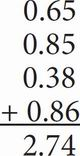# SAT Math Multiple Choice Question 9: Answer and Explanation

### Test Information

Question: 9

5. If each number in the following sum were increased by t, the new sum would be 4.22. What is the value of t ?• A. 0.24
• B. 0.29
• C. 0.33
• D. 0.37